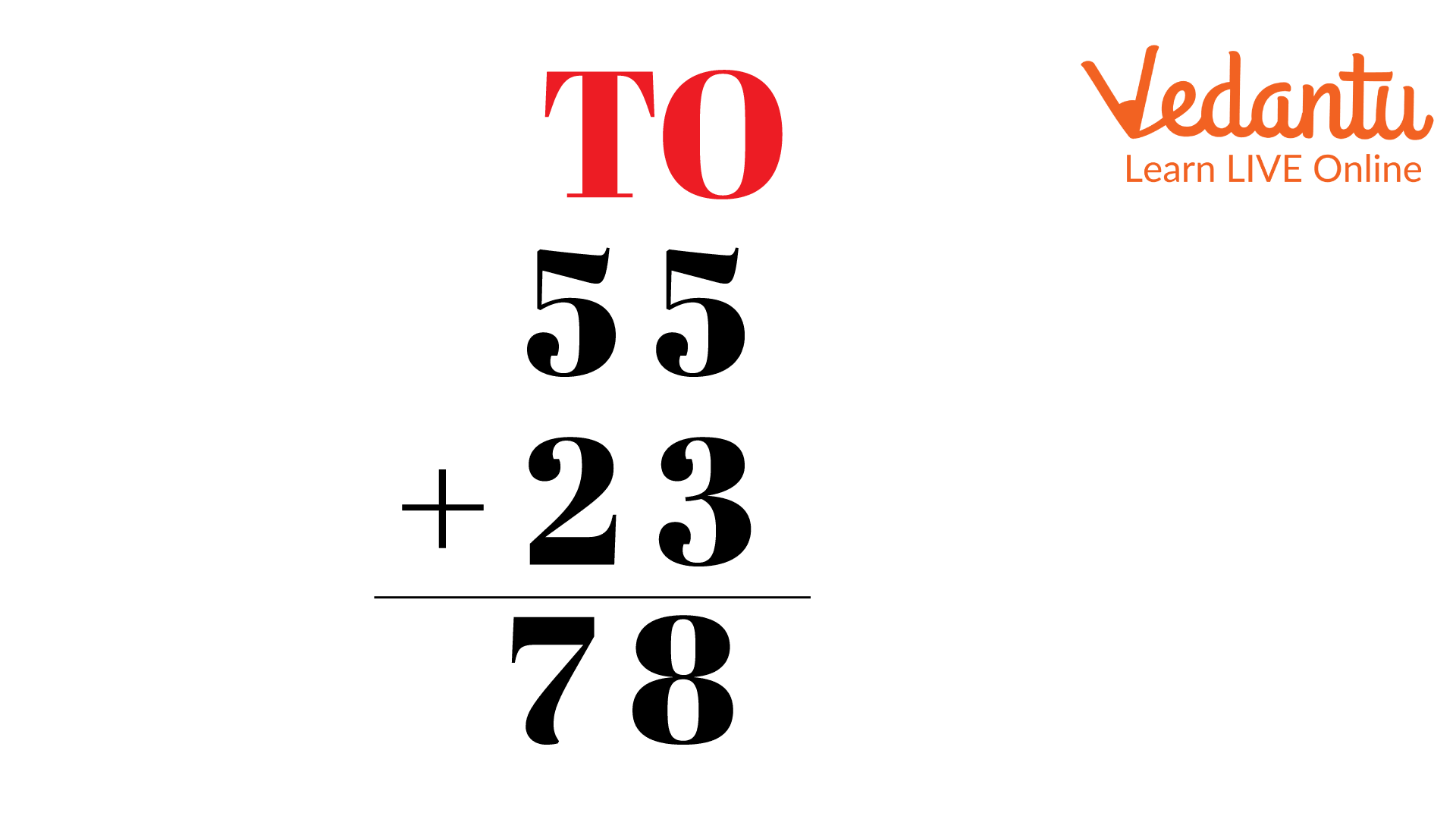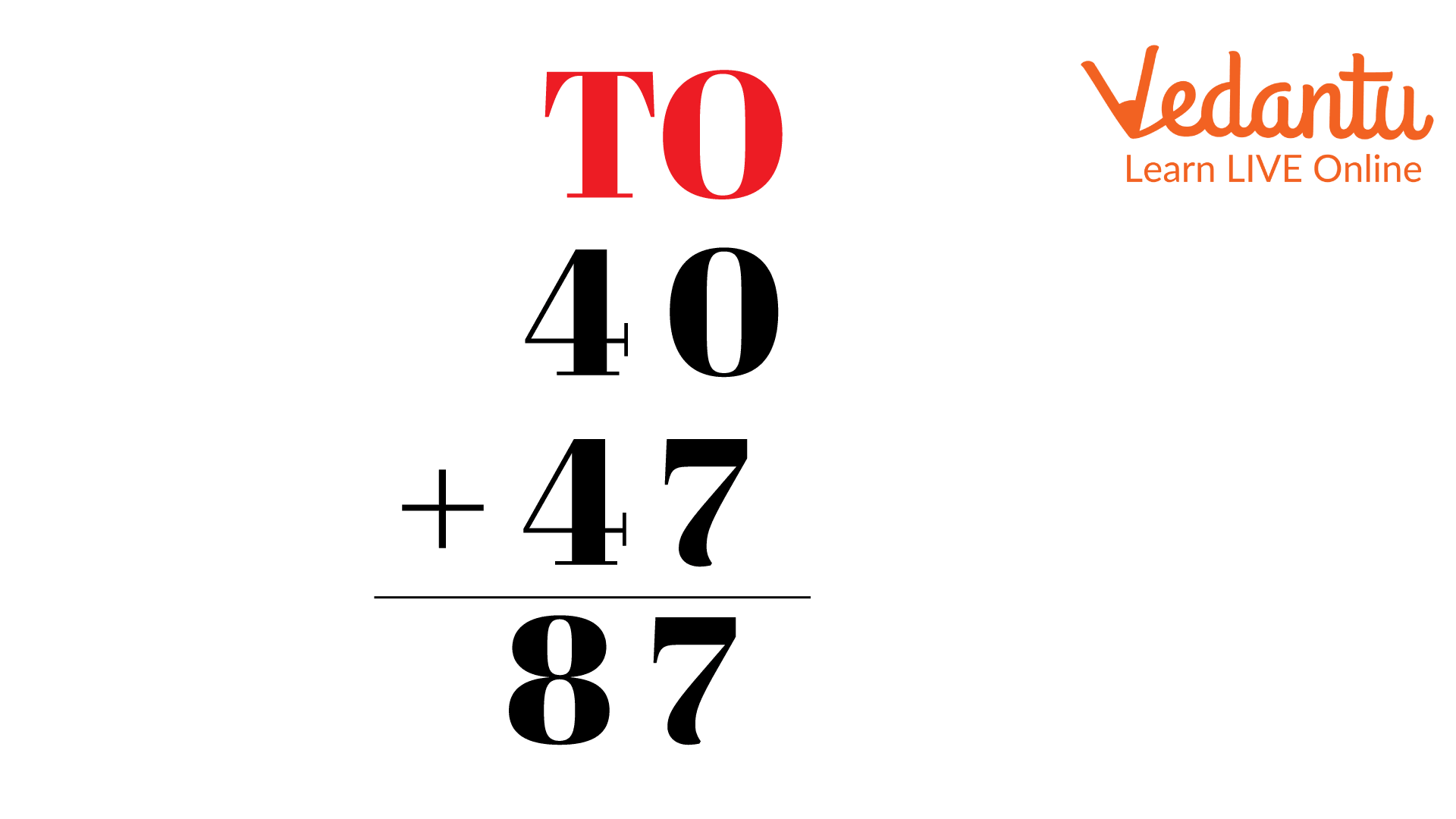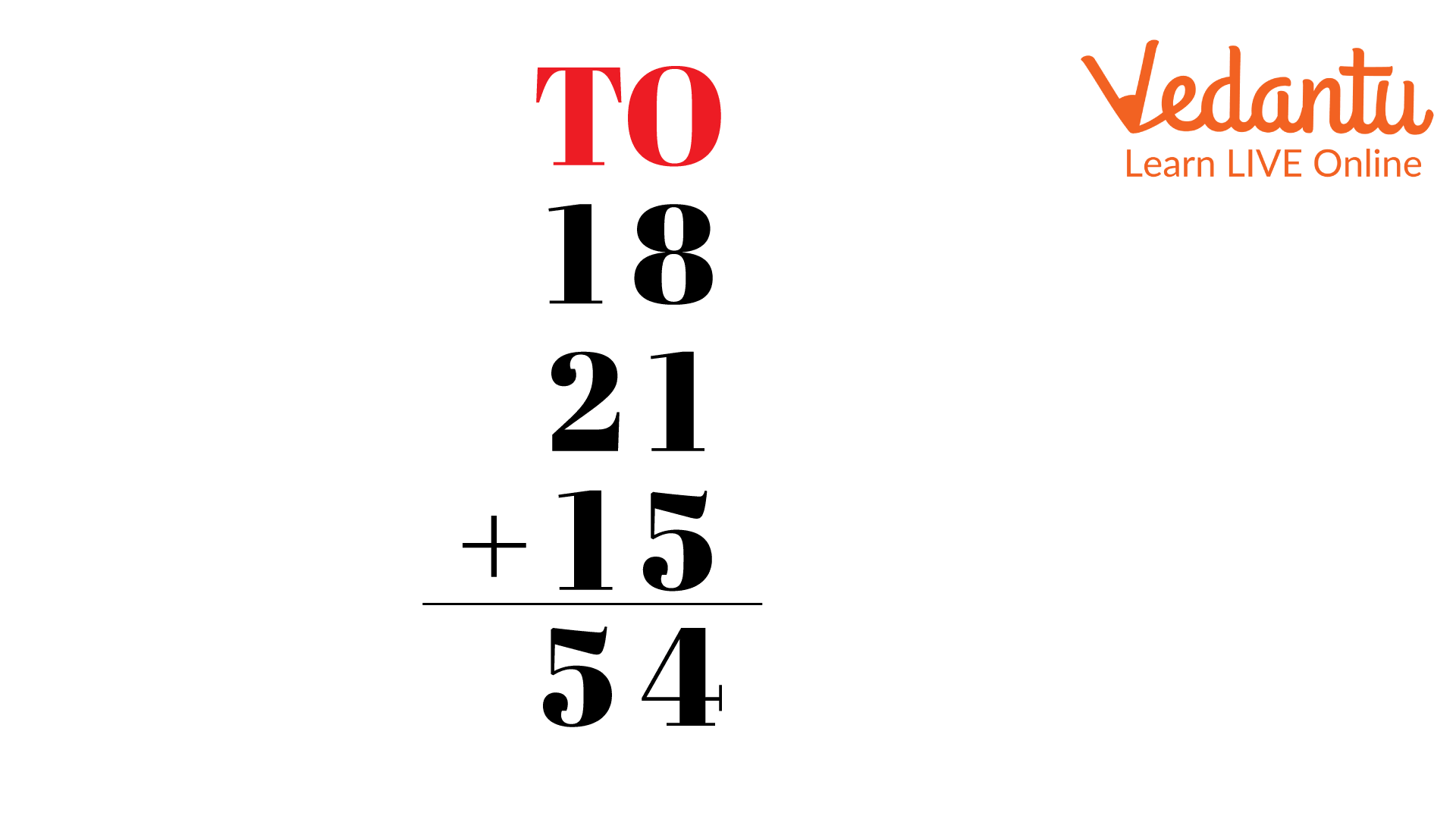Courses
Courses for Kids
Free study material
Free LIVE classes
MoreLIVE
Join Vedantu’s FREE Mastercalss

## Introduction

Without first having a working knowledge of addition, 2-Digit Addition is not possible. It is a method of adding something to another.

In fact, two-digit addition involves adding two or more numbers that have two digits each. The result is referred to as the two numbers added together.

To find a sum, the addends are added to each other. The amount can also be referred to as the "total." Today's discussion will focus on two-digit addition word problems.We know the four basic mathematical operations, i.e., addition, subtraction, multiplication and division. It is an operation of adding two or more numbers together.

Like any other basic mathematic operation, addition also has a unique symbol, i.e., ‘+’.

For example, if Sima ate 55 apples and 23 oranges. Then the total number of fruits that she ate $\begin{array}{l} = 55 + 23 = 78\end{array}$ fruits.55 + 23

## Addition Sums for Class 2

Let us solve some word problems on addition.

Example 1: Rishi received 36 boxes of candy and 12 cartons of canned noodles during the first week. He received 24 boxes of candy and 25 boxes of canned noodles during the second week. How many boxes of candy did he receive in total?

In the first week, the no. of candy boxes Rishi received $= 36$ and the no. of canned noodles $= 12$.

In the second week, the no. of boxes of candy Rishi received $= 24$ and the no. of canned noodles $= 25$.

According to the question,36+24

Total no. of boxes of candy that Rishi received $\begin{array}{l} = 36 + 24 = 60\end{array}$.

Therefore, the total number of boxes of candy he received is 60.

Example 2: 40 boxes were used to hold the juice, and 47 more were brought last month. How many juice boxes are there in total?

No. of boxes of juice that were stored $= 40$ and no. of boxes that were delivered last month $= 47$.

According to the question,47+40

Total no. of juice boxes $\begin{array}{l} = 40 + 47 = 87\end{array}$

Therefore, the total no. of juice boxes is 87.

Example 3: Rishi went to school for 18 and 21 days in January and February, respectively. What will be his total attendance if he goes to school for 15 days in March?

Rishi went to school in January $= 18$ days, in February $= 21$ days and in March $= 15$ days.

According to the question,18 + 21 + 15

Rishi total attendance $\begin{array}{l} = 18 + 21 + 15~{\rm{ days}} {\rm{ = 54~days}}\end{array}$

Therefore, the total attendance of Rishi is 54 days.

Let us look at some 2-Digit addition worksheets.

Example 1: Ani purchased two water jugs. Each jug has a capacity of 5 and 10 gallons of water, respectively. How much water, in total, can the two jugs hold?

Capacity of first jug $= 5$ gallons and capacity of second jug $= 10$ gallons.

According to the question,10 + 5

Total capacity of the two jugs $\begin{array}{l} = 5 + 10~{\rm{gallons}}~{\rm{ = 15~gallons}}\end{array}$

Therefore, the capacity of the water that the two jugs can hold is 15 gallons.

Example 2: Megha gathered 12 lettuces and 10 cabbages from her kitchen garden. How many vegetables are in total?

No. of lettuces that Megha gathered = 12 and no. of cabbages that Megha gathered = 10.

According to the question,

Total no. of vegetables $\begin{array}{l} = 12 + 10 = 22\end{array}$

Therefore, the total no. of vegetables that Megha gathered is 22.

Example 3: The flight attendant loads 15 boxes of cookies, 25 cans of cola, and 70 cans of fruit juice into the catering cart. How many cans of drinks are there in total?

No. of cookies $= 15$, no. of cola cans $= 25$ and no. of fruit juice $= 70$.

According to the question,

The total no. of cans of drinks $\begin{array}{l} = 25 + 70 = 95\end{array}$

Therefore, the total no. of cans of drinks is 95.

## Conclusion

A kid can start learning about numbers by counting small groups of items as early as 12 months. At 3 to 4 years old, though, we begin with the basics of addition and work our way up to the more difficult parts. Then we start to understand that we can quickly find the total number of objects using addition. Whether in markets or institutions for money transactions, we use addition.

Last updated date: 01st Oct 2023
Total views: 69.3k
Views today: 2.69k

## FAQs on 2-Digit Addition Word Problems

1. How can a child learn 2-digit addition?

It can be done using the digital open number line method. For better understanding, if a child is adding 25 and 43, the child will start at one of the numbers on the number line: 25. Then, add the tens: 40, making it 65. Finally, adding the ones: 3, coming to their answer, 68.

2. What is a two-step word problem?

A word problem is a few sentences presenting a "real-life" situation where an issue needs to be solved using math. It can be solved in two steps, known as a two-step problem.

3. What comes first in a word problem solution?

Reading a Math word problem through to the end will help us understand what needs to be done to solve it. After reading it, we can quickly tell which parts of the problem need to be solved and which parts give information regarding the problem.